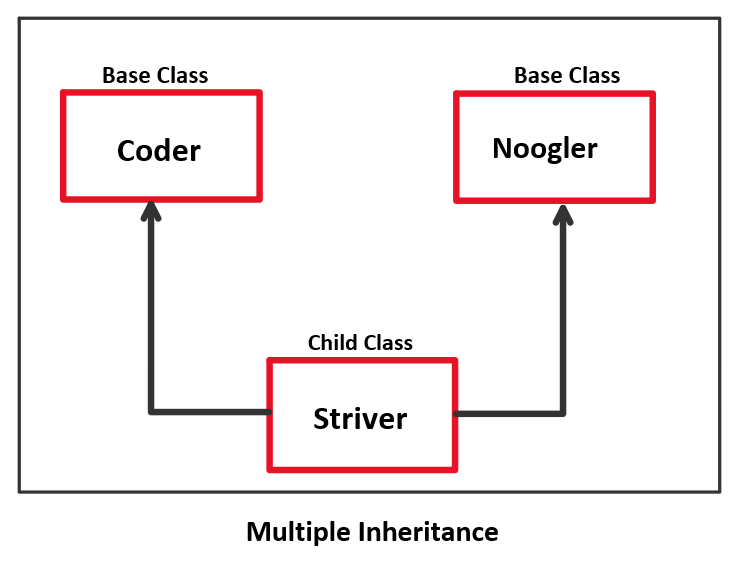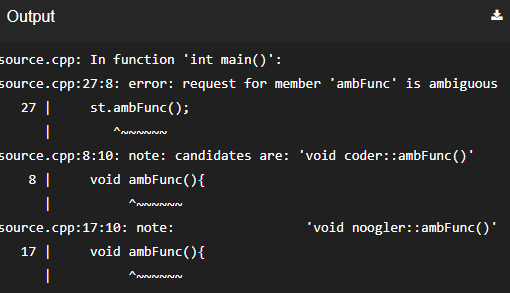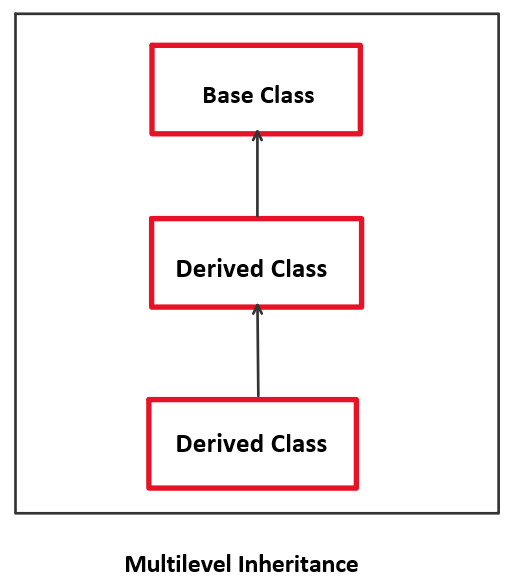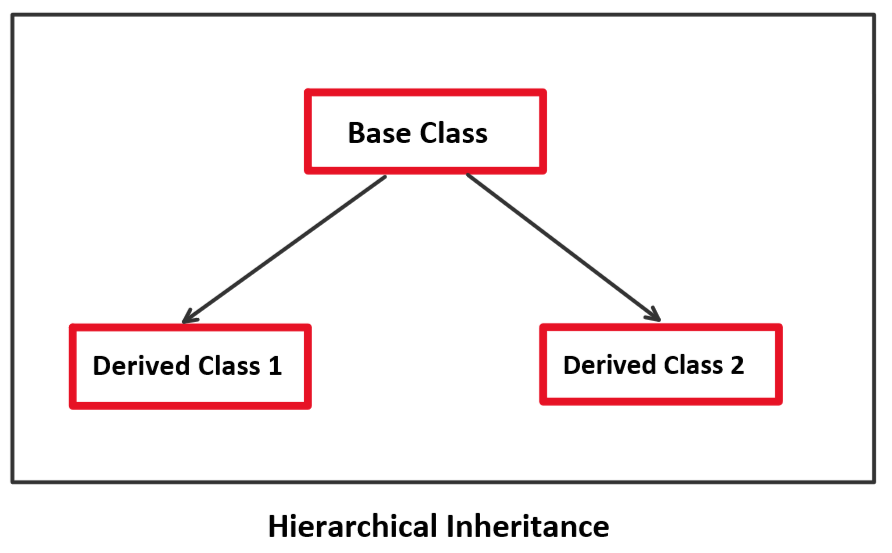# Inheritance in C++ : Multiple, Multilevel, hierarchical

### Introduction

C++ has various OOP concepts which also include inheritance.

Inheritance allows us to create a new class from an existing class by copying the members of the existing class and using them in the new class. Here the new class is also known as the derived class/ child class whereas the existing class is also known as the parent class.

There are various types of inheritance but in this article, we would discuss the following three types:

1. Multiple Inheritance
2. Multilevel Inheritance
3. Hierarchical Inheritance

### Multiple Inheritance

In this type of inheritance, there may exist multiple parents for a single derived class.

Let’s take an example, Striver is also a coder and Noogler. Here coder and noogler work as parent class and striver himself work as a derived/child class.

The following diagram gives a better understanding of this.Let’s understand it with the code

Code:

## C++ Code

``````#include <iostream>
using namespace std;

class coder {
public:
void printCoder() {
cout << "I'm also a coder" << "\n";
}
};
class noogler {
public:
void printNoogler() {
cout << "I'm also a noogler" << "\n";
}
};
class striver: public noogler, public coder {};

int main() {
striver st;
st.printNoogler();
st.printCoder();
}
``````

Output:

I’m also a noogler
I’m also a coder

Ambiguity in Multiple Inheritance

When performing multiple inheritances, it is possible that the base classes have the same function names and if the derived class tries to access that function, ambiguity arises as the compiler gets confused about which base’s class function to be called and executed.

Let’s use the above example and try to understand this topic

Code:

## C++ Code

``````#include <iostream>
using namespace std;
class coder {
public:
void ambFunc() {
cout << "I'll raise ambiguity" << "\n";
}
};
class noogler {
public:
void ambFunc() {
cout << "I'll raise ambiguity" << "\n";
}
};
class striver: public noogler, public coder {};

int main() {
striver st;
st.ambFunc(); // error
}
``````

output:Now, how to resolve this issue – We can use the scope resolution (::) operator. The following snippet explains the solution.

```st.coder::ambFunc();
st.noogler::ambFunc();
```

### Multilevel Inheritance

In this type of inheritance, you can try deriving a class from a derived class. It’s like

Base class -> Derived class -> Derived Class

Let’s try visualizing it with the help of a diagramThinking of a practical example for this approach, you can say – Scooty, Honda, Activa. Scooty works like the base class and Honda comes under that scooty class which works like a derived class and Activa coming under that honda class acts like the sub derived class.

Now let’s try coding this approach

Code:

## C++ Code

``````#include <iostream>
using namespace std;

class Scooty {
public:
void print() {
cout << "Scooty class" << "\n";
}
};
class Honda: public Scooty {};
class Activa: public Honda {};

int main() {
Activa ac;
ac.print(); // prints Scooty Class
}
``````

Output: Scooty class

Hierarchical Inheritance

If more than one class is derived from the base class, this results in hierarchical inheritance. You can assume the diagram of this hierarchical inheritance as a family tree.
Let’s try visualizing it with the help of a diagram.Now let’s try understanding it with the code

Code:

## C++ Code

``````#include <iostream>
using namespace std;

// base class
class Striver {
public:
void pStriver() {
cout << "Hi, I'm striver" << "\n";
}
};
// derived class 1
class youtuber: public Striver {
public: void pVlog() {
cout << "This is my vlogging channel, striver" << "\n";
}
};
// derived class 2
class educator: public Striver {
public: void pTUF() {
cout << "This is my edu channel, takeuforward" << "\n";
}
};

int main() {

// derived class 1 object
y.pStriver(); // base class function
y.pVlog();

// derived class 2 object
educator e;
e.pStriver(); // base class function
e.pTUF();
}
``````

Output:

Hi, I’m striver
This is my vlogging channel, striver
Hi, I’m striver
This is my edu channel, takeuforward

Special thanks to Yash Mishra for contributing to this article on takeUforward. If you also wish to share your knowledge with the takeUforward fam, please check out this article